# MAP 7th Grade Math : The Real and Complex Number Systems

## Example Questions

### Example Question #1 : The Real And Complex Number Systems

If a server at a restaurant gets atip on a bill of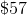, then what will be the amount of the tip?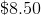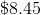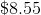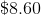Explanation:

The first step to solving this problem is determining what operation we are going to use.

Think:we know that the tip is going to beof.  The keyword "of" gives us a clue and indicates that we are going to multiply.

As we have learned in previous lessons, we need to convert our percentage into a decimal before we are able to multiply: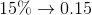Next, we can perform the multiplication operation and find the tip: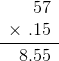The server's tip will be### Example Question #1 : The Real And Complex Number Systems

Solve: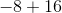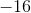Explanation:

In order to solve this problem, we need to start aton the number line.Next, we have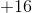which means we need to moveplaces to the right on the number line. When we have an addition sign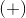we move to the right because that is towards the positive side of the number line. When we have a subtraction sign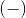we move to the left because that is towards the negative side of the number line.The orange arrow movedplaces to the right, and ended at; thus,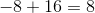### Example Question #1 : The Real And Complex Number Systems

Does the table provided show a direct proportional relationship? If so, then identify the proportion.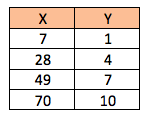No

Yes,Yes,Yes,Yes,Explanation:

In order to determine whether or not the table shows a direct proportion, we will divide the quantities in thecoordinate column by the quantities in the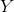coordinate column. In order for the table to show direct proportion, each quotient should be the same value.

In this example, each quotient is the same value; therefore, this table does show direct proportion.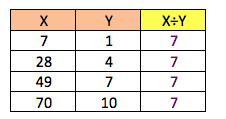The proportion is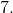### All MAP 7th Grade Math Resources